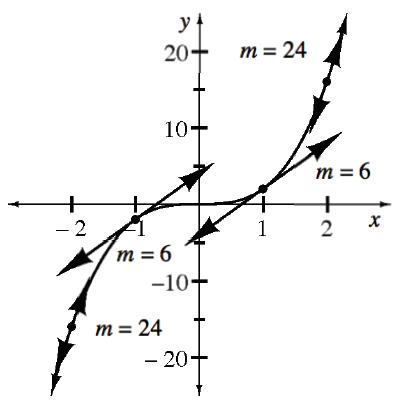### Home > APCALC > Chapter 3 > Lesson 3.1.1 > Problem3-11

3-11.

Below is a graph of the function $f(x) = 2x^3$ with tangent lines drawn at $x = −2, −1, 1,$ and $2$. Use the slopes provided in the graph to determine the slope function $f^\prime$. Notice that $f^\prime (0) = 0$. It might be helpful to make a table of data relating $x$ to $m$

The data in the table was taken from the graph.
'$x$' represents $x$-values.
'$m$' represents slope of the tangent line.

 $x$ $–2$ $–1$ $0$ $1$ $2$ $m$ $24$ $6$ $0$ $6$ $24$

The original graph, $f(x)$, is cubic. Does the table of slopes also appear to have a cubic pattern? If not, what type of function would model its pattern?

The slopes have a quadratic pattern! Clearly, the data does not fit the parent quadratic equation: $y = x^2$. Find a transformation of $y = x^2$ that models the data.Use the eTool below to view the tangent lines.
Click on the link to the right to view the full version of the eTool: Slope at a Point eTool